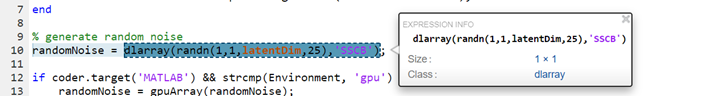## `dlarray` Limitations for Code Generation

### Recommended Usage

For code generation, use the `dlarray` (Deep Learning Toolbox) function to create deep learning arrays. For example, suppose you have a pretrained `dlnetwork` (Deep Learning Toolbox) network object in the `mynet.mat` MAT-file. To predict the responses for this network, create an entry-point function in MATLAB® as shown in this code.

```function a = foo(in) dlIn = dlarray(in, 'SSC'); persistent dlnet; if isempty(dlnet) dlnet = coder.loadDeepLearningNetwork('mynet.mat'); end dlA = predict(dlnet, dlIn); a = extractdata(dlA); end ```

### Limitations

For deep learning arrays, code generation has the following limitations:

• The data format argument of the `dlarray` object must be a compile-time constant. For example,

```function out = foo() dlA = dlarray(ones(5,4),'SSC'); %fmt 'SSC' is constant . . . end```

• The data input to the `dlarray` object must be fixed-size. For example, the `dlarray` `dlA` is not supported as `A` is variable-sized.

```function dlA = foo() A = ones(5,4); coder.varsize('A') %'A' is variable sized. dlA = dlarray(A, 'SSC'); % Error: not supported. end ```

• Code generation does not support creating a `dlarray` type object by using the `coder.typeof` function with upper bound size and variable dimensions specified. For example,

```function dlA = foo() A = dlarray(ones(5,4),'SC'); A_type = coder.typeof(A,[5 10],[1 0]); % Error: not supported. end ```

Code generation supports use of `coder.typeof` without the size arguments. For example,

```A = dlarray(ones(5,4),'SC'); A_type = coder.typeof(A); ```

• The code generation report does not display the size of the `dlarray` object. The size is always displayed as `1x1`.• In MATLAB, `dlarray` enforces the order of labels `'SCBTU'`. This enforcement eliminates ambiguous semantics in operations, which implicitly match labels between inputs. This behavior is mimicked during MEX code generation. However, for standalone code generation such as static, dynamic libraries, or executables, the data format follows the specification of the `fmt` argument of the `dlarray` object. As a result, if the input or output of an entry-point function is a `dlarray` object and its order of labels is not `'SCBTU'`, then the data layout will be different between the MATLAB environment and standalone code.

For example, consider a function `foo` with a `dlarray` object as an output.

```function dlA = foo() rng default dlA = dlarray(rand(5,4), 'BC'); end ```

In MATLAB, `dlA` is `4(C)`-by-`5(B)`.

```dlA = 4(C) × 5(B) dlarray 0.8147 0.9058 0.1270 0.9134 0.6324 0.0975 0.2785 0.5469 0.9575 0.9649 0.1576 0.9706 0.9572 0.4854 0.8003 0.1419 0.4218 0.9157 0.7922 0.9595 ```

For standalone code generation, `dlA` is `5(B)`-by-`4(C)`.

• For code generation, the `dlarray` input to the `predict` method of the `dlnetwork` object must be `single` data type.

## Related Examples